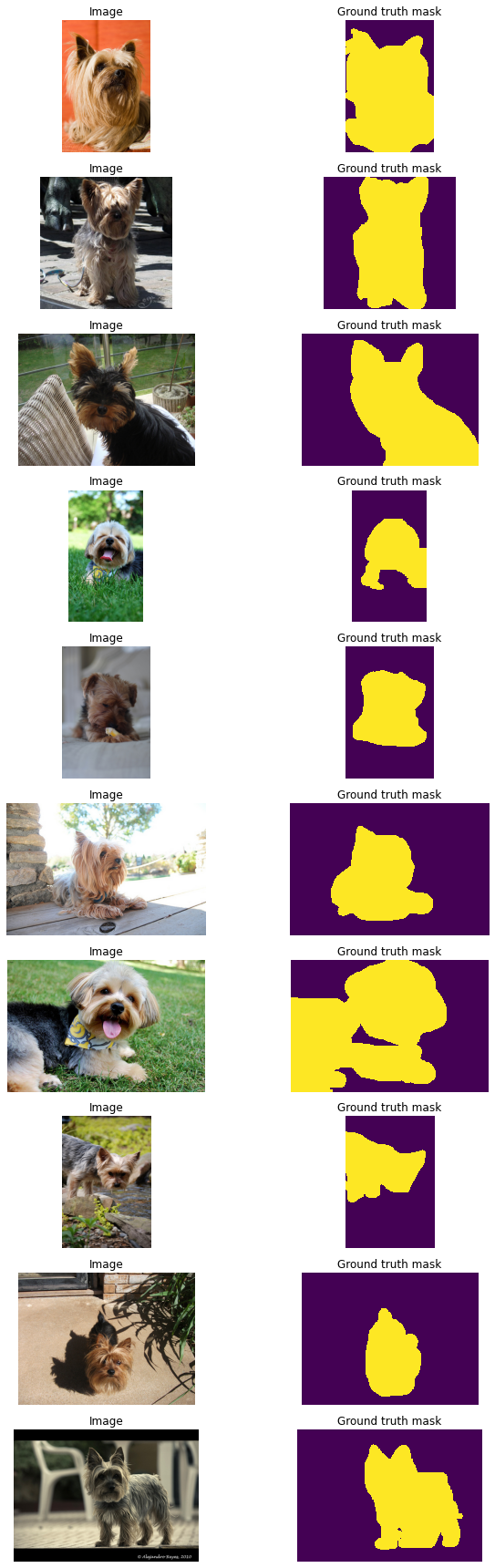# PyTorch and Albumentations for semantic segmentation¶

This example shows how to use Albumentations for binary semantic segmentation. We will use the The Oxford-IIIT Pet Dataset . The task will be to classify each pixel of an input image either as `pet` or `background`.

### Install the required libraries¶

We will use TernausNet, a library that provides pretrained UNet models for the semantic segmentation task.

``````!pip install ternausnet > /dev/null
``````

### Import the required libraries¶

``````from collections import defaultdict
import copy
import random
import os
import shutil
from urllib.request import urlretrieve

import albumentations as A
import albumentations.augmentations.functional as F
from albumentations.pytorch import ToTensorV2
import cv2
import matplotlib.pyplot as plt
import numpy as np
import ternausnet.models
from tqdm import tqdm
import torch
import torch.backends.cudnn as cudnn
import torch.nn as nn
import torch.optim

cudnn.benchmark = True
``````

``````class TqdmUpTo(tqdm):
def update_to(self, b=1, bsize=1, tsize=None):
if tsize is not None:
self.total = tsize
self.update(b * bsize - self.n)

directory = os.path.dirname(os.path.abspath(filepath))
os.makedirs(directory, exist_ok=True)
if os.path.exists(filepath):
return

with TqdmUpTo(unit="B", unit_scale=True, unit_divisor=1024, miniters=1, desc=os.path.basename(filepath)) as t:
urlretrieve(url, filename=filepath, reporthook=t.update_to, data=None)
t.total = t.n

def extract_archive(filepath):
extract_dir = os.path.dirname(os.path.abspath(filepath))
shutil.unpack_archive(filepath, extract_dir)
``````

``````dataset_directory = os.path.join(os.environ["HOME"], "datasets/oxford-iiit-pet")
``````

### Download and extract the `Cats vs. Docs` dataset¶

``````filepath = os.path.join(dataset_directory, "images.tar.gz")
url="https://www.robots.ox.ac.uk/~vgg/data/pets/data/images.tar.gz", filepath=filepath,
)
extract_archive(filepath)
``````
``````Dataset already exists on the disk. Skipping download.
```
```
``````filepath = os.path.join(dataset_directory, "annotations.tar.gz")
url="https://www.robots.ox.ac.uk/~vgg/data/pets/data/annotations.tar.gz", filepath=filepath,
)
extract_archive(filepath)
``````
``````Dataset already exists on the disk. Skipping download.
```
```

### Split files from the dataset into the train and validation sets¶

Some files in the dataset are broken, so we will use only those image files that OpenCV could load correctly. We will use 6000 images for training, 1374 images for validation, and 10 images for testing.

``````root_directory = os.path.join(dataset_directory)
images_directory = os.path.join(root_directory, "images")

images_filenames = list(sorted(os.listdir(images_directory)))
correct_images_filenames = [i for i in images_filenames if cv2.imread(os.path.join(images_directory, i)) is not None]

random.seed(42)
random.shuffle(correct_images_filenames)

train_images_filenames = correct_images_filenames[:6000]
val_images_filenames = correct_images_filenames[6000:-10]
test_images_filenames = images_filenames[-10:]

print(len(train_images_filenames), len(val_images_filenames), len(test_images_filenames))
``````
``````6000 1374 10
```
```

### Define a function to preprocess a mask¶

The dataset contains pixel-level trimap segmentation. For each image, there is an associated PNG file with a mask. The size of a mask equals to the size of the related image. Each pixel in a mask image can take one of three values: `1`, `2`, or `3`. `1` means that this pixel of an image belongs to the class `pet`, `2` - to the class `background`, `3` - to the class `border`. Since this example demonstrates a task of binary segmentation (that is assigning one of two classes to each pixel), we will preprocess the mask, so it will contain only two uniques values: `0.0` if a pixel is a background and `1.0` if a pixel is a pet or a border.

``````def preprocess_mask(mask):
``````

### Define a function to visualize images and their labels¶

Let's define a visualization function that will take a list of images' file names, a path to the directory with images, a path to the directory with masks, and an optional argument with predicted masks (we will use this argument later to show predictions of a model).

``````def display_image_grid(images_filenames, images_directory, masks_directory, predicted_masks=None):
cols = 3 if predicted_masks else 2
rows = len(images_filenames)
figure, ax = plt.subplots(nrows=rows, ncols=cols, figsize=(10, 24))
for i, image_filename in enumerate(images_filenames):
image = cv2.cvtColor(image, cv2.COLOR_BGR2RGB)

ax[i, 0].imshow(image)

ax[i, 0].set_title("Image")

ax[i, 0].set_axis_off()
ax[i, 1].set_axis_off()

ax[i, 2].set_axis_off()
plt.tight_layout()
plt.show()
``````
``````display_image_grid(test_images_filenames, images_directory, masks_directory)
``````### Image sizes for training and prediction¶

Often, images that you use for training and inference have different heights and widths and different aspect ratios. That fact brings two challenges to a deep learning pipeline: - PyTorch requires all images in a batch to have the same height and width. - If a neural network is not fully convolutional, you have to use the same width and height for all images during training and inference. Fully convolutional architectures, such as UNet, can work with images of any size.

There are three common ways to deal with those challenges: 1. Resize all images and masks to a fixed size (e.g., 256x256 pixels) during training. After a model predicts a mask with that fixed size during inference, resize the mask to the original image size. This approach is simple, but it has a few drawbacks: - The predicted mask is smaller than the image, and the mask may lose some context and important details of the original image. - This approach may be problematic if images in your dataset have different aspect ratios. For example, suppose you are resizing an image with the size 1024x512 pixels (so an image with an aspect ratio of 2:1) to 256x256 pixels (1:1 aspect ratio). In that case, this transformation will distort the image and may also affect the quality of predictions. 2. If you use a fully convolutional neural network, you can train a model with image crops, but use original images for inference. This option usually provides the best tradeoff between quality, speed of training, and hardware requirements. 3. Do not alter the sizes of images and use source images both for training and inference. With this approach, you won't lose any information. However, original images could be quite large, so they may require a lot of GPU memory. Also, this approach requires more training time to obtain good results.

Some architectures, such as UNet, require that an image's size must be divisible by a downsampling factor of a network (usually 32), so you may also need to pad an image with borders. Albumentations provides a particular transformation for that case.

The following example shows how different types of images look.

``````example_image_filename = correct_images_filenames
image = cv2.cvtColor(image, cv2.COLOR_BGR2RGB)

resized_image = F.resize(image, height=256, width=256)
cropped_image = F.center_crop(image, crop_height=256, crop_width=256)
``````
``````figure, ax = plt.subplots(nrows=1, ncols=5, figsize=(18, 10))
ax.ravel().imshow(image)
ax.ravel().set_title("Original image")
ax.ravel().imshow(resized_image)
ax.ravel().set_title("Resized image")
ax.ravel().imshow(cropped_image)
ax.ravel().set_title("Cropped image")